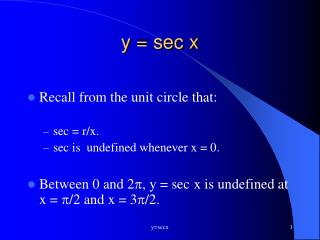# y = sec x - PowerPoint PPT PresentationDownload Presentationy = sec x

y = sec x
Download Presentation## y = sec x

- - - - - - - - - - - - - - - - - - - - - - - - - - - E N D - - - - - - - - - - - - - - - - - - - - - - - - - - -
##### Presentation Transcript

1. y = sec x • Recall from the unit circle that: • sec = r/x. • sec is undefined whenever x = 0. • Between 0 and 2, y = secx is undefined at x = /2 and x = 3/2. y=secx

2. Domain • Since sec x is undefined at /2, 3/2, etc., the asymptotes appear at /2 + k y=secx

3. Range of Secant Function • The range of every secant graph varies depending on vertical shifts. • The range of the parent graph is (-, -1]U[1, ) y=secx

4. Period • One complete cycle occurs between 0 and 2. • The period is 2. y=secx

5. Critical Points • The parent graph has a local maximum at (, -1). • The parent graph has a local minimum at (0, 1) and (2,1). y=secx

6. y = a sec b(x-c) +d • a = vertical stretch or shrink • If |a| > 1, the graph has a vertical stretch . • If 0<|a|<1, the graph has a vertical shrink . • If a is negative, the graph reflects about the x-axis. y=secx

7. y = a sec b (x - c) +d • b= horizontal stretch or shrink • Period = 2/b • If |b| >1, the graph contains a horizontal shrink . • If 0<|b|<1, the graph contains a horizontal stretch. y=secx

8. y = a sec b(x - c ) +d • c= horizontal shift • if c is negative, the graph shifts left c units. • if c is positive, the graph shifts right c units. y=secx

9. y = a sec b(x - c) + d • d= vertical shift • If d is positive, the graph shifts up d units. • If d is negative, the graph shifts down d units y=secx

10. Parent Function: y = sec x • Important Points: • : asymptote • Important Points: • : asymptote • Important Points: • : asymptote y=secx

11. Graph: y = sec x y=secx

12. Graph: y = 3 sec x y=secx

13. Graph: y = sec ½ x y=secx

14. Graph: y=secx

15. Graph: y = sec x - 3 y=secx

16. Graph: y=secx